﻿

Aruler and segment-transporter constructive axiomatization of plane hyperbolic geometry

Abstract

We formulate a universal axiom system for plane hyperbolic geometry in a first-order language with one sort of individual variables, points (lower-case), containing three individual constants,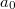$a_0$,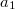$a_1$,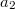$a_2$, standing for three non-collinear points, with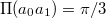$\Pi(a_0a_1)=\pi/3$, one quaternary operation symbol$\tilde{\iota}$, with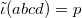$\tilde{\iota}(abcd)=p$ to be interpreted as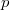$p$ is the point of intersection of lines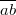$\overline{ab}$ and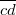$\overline{cd}$, provided that lines$\overline{ab}$ and$\overline{cd}$ are distinct and have a point of intersection, an arbitrary point, otherwise', and two ternary operation symbols,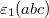$\varepsilon_1(abc)$ and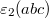$\varepsilon_2(abc)$, with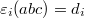$\varepsilon_{i}(abc)=d_i$ (for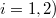$i=1,2)$ to be interpreted as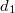$d_1$ and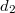$d_2$ are two distinct points on line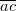$\overline{ac}$ such that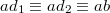$ad_1 \equiv ad_2 \equiv ab$, provided that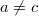$a\not=c$, an arbitrary point, otherwise'.

DOI Code: 10.1285/i15900932v22n1p1

Keywords: Hyperbolic geometry; Constructive axiomatization; Quantifier-free axiomatization

Full Text: PDF